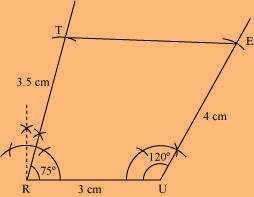1. If the four sides and a diagonal of the quadrilateral are given.

ExampleConstruct a quadrilateral ABCD in which AB   =   5 cm, BC   =   7 cm, CD   =   6 cm, DA = 6.5 cm  and  AC  =  8 cm.

Step 1:  ∆ ABC can be constructed using S.S.S criterion of the construction of triangle.

Step 2: Here  AC is the diagonal, so D will be somewhere opposite to B with reference to AC.

and  AD  = 6.5 cm so draw an arc from A as the centre with radius  6.5 cm.

Step 3: Now draw an arc with C as the centre and by taking radius 6 cm. This arc  should

intersect the above arc.

Step 4: The point of intersection of the two arcs is point D. Now join AD and DC to complete the quadrilateral.

Hence, ABCD is the required quadrilateral.

EXERCISE:   4.1

(i)        Quadrilateral ABCD where  AB   =   4.5 cm, BC   =   5.5 cm,  CD   =   4 cm,

AD   =   6 cm,   AC   =   7 cm

(ii)       Quadrilateral JUMP where        JU   =  3.5 cm, UM   =   4 cm,  MP   =   5 cm,

PJ   =  4.5 cm,  PU   =   6.5 cm

(iii)     Parallelogram MORE where   OR   =   6 cm, RE   =   4.5 cm, EO   =   7.5 cm

(iv)      Rhombus BEST where   BE   =   4.5 cm, ET   =   6 cm

(i)        Given:  AB   = 4.5 cm,  BC  = 5.5 cm,  CD  =  4 cm,  AD  =  6 cm,  AC  =  7 cm

Steps of construction:

(a)   Draw AB   =   4.5 cm.

(b)   Draw an arc taking radius 5.5 cm from point B.

(c)   Taking radius 7 cm, draw an another arc from point A which intersects the first arc at point C.

(d)   Join BC and AC.

(e)   Draw an arc of radius 6 cm from point A and draw another arc of radius 4 cm from point C which intersects at D.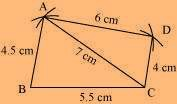(ii) Given:  JU  =  3.5 cm,   UM  =  4 cm,  MP  =  5 cm,  PJ  =  4.5 cm,  PU  =  6.5 cm

Steps of construction:

(a)  Draw JU   =   3.5 cm.

(b)  Draw an arc of radius 4.5 cm taking centre J and then draw another arc of radius 6.5 cm taking U as centre. Both arcs intersect at P.

(c)  Join PJ and PU.

(d) Draw arc of radius 5 cm and 4 cm taking P and U as centres respectively, which intersect at M.

(e)  Join Mp and MU.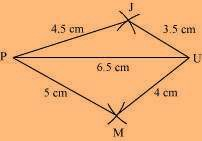(iii) Given:  OR  =  6 cm,  RE  =  4.5 cm,   EO  =  7.5 cm

To construct: A parallelogram MORE.

Steps of construction:

(a)   Draw OR   =   6 cm.

(b)   Draw arcs of radius 7.5 cm and radius 4.5 cm taking O and R as centres respectively, which

intersect at E.

(c ) Join OE and RE.

(d)   Draw an arc of 6 cm radius taking E as center.

(e)   Draw another arc of 4.5 cm radius taking O as center, which intersects at M.

(f)   Join OM and EM.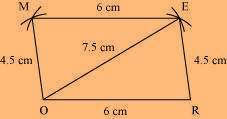The  required parallelogram  is MORE.

(iv)   Given:  BE  =  4.5 cm,  ET  =  6 cm

To construct: A rhombus BEST.

Steps of construction:

(a)   Draw TE   =   6 cm and bisect it into two equal parts.

(b)   Draw up and down perpendiculars to TE.

(c)   Draw two arcs of  4.5 cm taking E and T as centers, which intersect at S.

(d)   Again draw two arcs of 4.5 cm taking E and T as centers, which intersects at B.

(e)   Join TS, ES, BT and EB.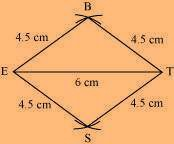The required rhombus  is BEST.

1. Construct a quadrilateral BEST, given ES =   4.5cm, BT  =   5.5 cm, St  =   5 cm, the diagonal BS  =   5.5 cm and diagonal ET  =   7 cm. Find Angle E, Angle T and RE.
2. Draw a line segment of length 10 cm and divide it into 4 equal parts.
3. Construct a quadrilateral PQRS with PQ =   4 cm, QR   =   3 cm, PS   =   2.5 cm, PR   =   4.5 cm and QS   =   4 cm

If  two diagonals and three sides of the quadrilateral are given:

Example:   Construct a quadrilateral ABCD if the two diagonals are AC =  6.5 cm and BD  =  8 cm. The other sides are BC  =  5.5 cm,  AD  = 6.5 cm and   CD  =  6 cm.

First of all, draw a rough sketch of the quadrilateral by using the given measurements. Then start constructing the real one.

Step 1: We can see that AD, AC and DC are given so we can construct a triangle Δ ACD by using S.S.S criterion.

Step 2: Now, BD is given so we can draw the point B keeping D as the centre and draw an arc of radius 8 cm just opposite to the point D with reference to AC.

Step 3: BC is given so we can draw an arc keeping C as centre and radius 5.5 cm so that it intersects the other arc.

Step 4: That point of intersection of the arcs is point B. Join AB and BC to complete the quadrilateral.

Thus  ABCD is the required quadrilateral.

EXERCISE 4.2

LI  =  4 cm,  IF  =  3 cm,  TL  =  2.5 cm,  LF  =  4.5 cm,  IT  =  4 cm

OL  =  7.5 cm,  GL  =  6 cm,  GD  =  6 cm,  LD  =  5 cm,  OD  =  10 cm

(iii) Rhombus BEND

BN  =  5.6 cm,  DE  =  6.5 cm

(i) Given:  LI  =  4 cm, IF  =  3 cm, TL  =  2.5 cm, LF  =  4.5 cm, IT  =  4 cm

Steps of construction:

(a)   Draw a line segment LI   =   4 cm.

(b)   Taking radius 4.5 cm, draw an arc taking L as center.

(c)   Draw an arc of 3 cm taking I as center which intersects the first arc at F.

(d)   Join FI and FL.

(e)   Draw another arc of radius 2.5 cm taking L as center and 4 cm taking I as center which

intersect at T.

(f) Join TF, Tl and TI.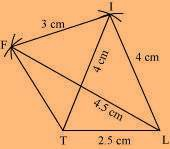(ii) Given:  OL   =   7.5 cm, GL   =   6 cm, GD   =   6 cm, LD   =   5 cm, OD   =   10 cm

Steps of construction:

(a)   Draw a line segment OL   =   7.5 cm

(b)   Draw an arc of radius 5 cm taking L as center and another arc of radius 10 cm taking O as

center which intersect the first arc point at D.

(c)   Join LD and OD.

(d)   Draw an arc of radius 6 cm from D and draw another arc of radius 6 cm taking L as center,

which intersects at G.

(e)   Join GD and GO.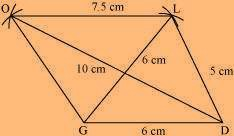(iii) Given:  BN   =   5.6 cm, DE   =   6.5 cm

To construct: A rhombus BEND.

Steps of construction:

(a)   Draw DE   =   6.5 cm.

(b)   Draw perpendicular bisector of line segment DE.

(c)   Draw two arcs of radius 2.8 cm from intersection point O, which intersects the line

KN at B   and N.

(d)   Join BE,  BD as well as  ND and NE.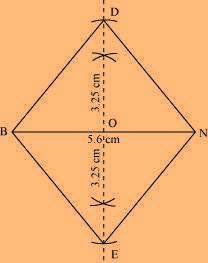The required rhombus   is BEND.

1. Construct a quadrilateral ABCD,  given that BC =   4.5 cm,   AD  =  5.5 cm,  CD  =  5 cm the diagonal AC  =  5.5 cm and diagonal  BD  =  7 cm.
2. Construct a quadrilateral DEFG,  given that EF =  5.5 cm,  DG  =  6.5 cm,  FG  =  5 cm the diagonal  DF  =  6.5 cm and diagonal EG  =  6.7 cm

1. If three angles and two adjacent sides of the quadrilateral are given.

Example: Construct a quadrilateral ABCD in which the two adjacent sides are

AB  =  4.5 cm and BC  =  7.5 cm. The given three angles are  ∠A  =  75ᵒ,  ∠B  =  105ᵒ and  ∠ C   =   120ᵒ.

Solution: Draw a rough sketch so that construction becomes easy.

Step 1: Draw AB  =  4.5 cm. Then measure  ∠B  =  105° using protractor and draw BC  =  7.5 cm.

Step 2: Draw  ∠ C  =  120°.

Step 3: Measure  ∠ A  =  75° and make a line until it touches the line coming from point C.

So ABCD is the required quadrilateral.

EXERCISE 4.3

MO   =   6 cm,   OR   =   4.5 cm,     ∠  M   =   60     ∠ O   =   105     ∠  R   =   105

PL   =   4 cm,   LA   =   6.5 cm,    ∠  P   =   90     A   =   110    N   =   85

(iii)     Parallelogram HEAR

HE   =   5 cm, EA   =   6 cm,  ∠ R   =   85

(iv)      Rectangle OKAY

OK   =   7 cm, KA   =   5 cm

(i) Given:  MO   =   6 cm,   OR   =   4.5 cm,    ∠ M   =   60    ∠ O   =   105     ∠ R   =   105

Steps of construction:

(a)   Draw a line segment MO  =  6 cm.

(b)   Construct  ∠O  =   105 and taking the  radius as 4.5 cm,  taking O as  centre, draw an arc  which   intersects at R.

(c)   Also construct an angle 105 at R and produce the side RE.

(d)   Construct another angle of 60 at point M and the side ME. Both  the sides ME and RE   intersect at E.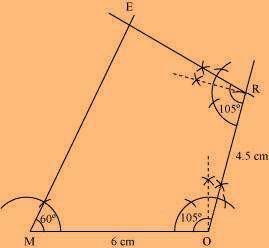(ii)       Given:  PL   =   4 cm, LA   =   6.5 cm,     ∠ P   =   90 ∠ A   =   110 ∠ N   =   85

To find:   ∠ L   =   360 – (90 +110   + 85 )

=   360   –  285  =  75

Steps of construction:

(a)   Draw a line segment PL   =   4 cm.

(b)   Construct angle of 90 at P and produce the side PN.

(c)   Construct angle of 75 at L and with L as center, draw an arc of radius 6 cm, which

intersects at A.

(d)   Construct  ∠ A   =   110 at A and produce the side AN which intersects PN at N.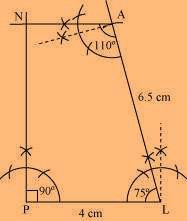(iii)     Given:  HE  =  5 cm, EA  =  6 cm,     ∠ R  =  85

To construct: A parallelogram HEAR.

To find:   ∠ H   =   180   –  85   = 95      ( Sum of adjacent angles of a parallelogram is 180)

Steps of construction:

(a)   Draw a line segment HE   =   5 cm.

(b)   Construct  ∠ H   =   95 and draw an arc of radius 6 cm with center H. It intersects AR at R.

(c)   Join RH.

(d)   Draw  ∠ R  =   ∠ E  =  85  and draw an arc of radius 6 cm with E as a centre which

intersects RA at A.

(e)   Join RA

The required parallelogram  is HEAR.

( iv)     Given:  OK   =   7 cm, KA   =   5 cm

To construct: A rectangle OKAY.

Steps of Construction:

(a)   Draw a line segment OK   =   7 cm.

(b)   Construct angle 90 at both points O and K and produce these sides.

(c)   Draw two arcs of radius 5 cm from points O and K respectively.

These arcs intersect at Y and A.

(d)   Join YA.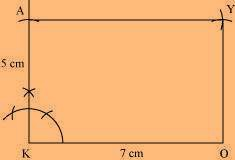The required rectangle  is OKAY.

1. Construct a quadrilateral ABCD in which AB =  5 cm, BC  =  6.5 cm, angle A  =   5°, angle B  =   105° and angle C  =  120°.
2. Construct a quadrilateral PQRS in which PQ =  7 cm, QR  =  7.5 cm, angle P  =  75°, angle Q  =  105° and angle R  =  120°.

If the three sides with two included angles of the quadrilateral are given.

Example:Construct a quadrilateral ABCD in which the three sides are AB  =  5 cm,

BC  =  6 cm and CD  = 7.5 cm. The two included angles are   B  =  105°  and   C   =   80°.

Solution:   Draw a rough sketch.

Step 1: Draw the line BC   =   6 cm. Then draw   ∠ B   =   105° and mark the length of AB   =   5 cm.

Step 2: Draw   ∠ C   =   80° using protractor towards point B.

Step 3: Mark the length of CD i.e.7.5 cm from C to make CD   =   7.5 cm.

Hence ABCD is the required quadrilateral.

EXERCISE 4.4

DE   =   4 cm, EA   =   5 cm, AR   =   4.5 cm,    ∠ E   =   60 ∠ A   =   90

TR   =   3.5 cm, RU   =   3 cm, UE   =   4 cm,      R   =   75 ∠ U   =   120

(i) Given:  DE   =   4 cm, EA   =   5 cm,  AR   =   4.5 cm,  ∠ E   =   60 ∠ A   =   90

Steps of construction:

(a)   Draw a line segment DE   =   4 cm.

(b)   At point E, construct an angle of 60

(c)   Taking radius 5 cm, draw an arc from point E which intersects at A.

(d)   Construct  ∠ A   =   90 draw an arc of radius 4.5 cm with center A which intersect at R.

(e)   Join RD.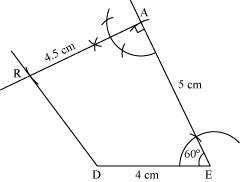(ii)       Given:  TR   =   3.5 cm,  RU   =   3 cm,   UE   =   4 cm,  ∠ R   = 75   ∠ U   =   120

Steps of construction:

(a)   Draw a line segment TR   =   3.5 cm.

(b)   Construct an angle 75 at R and draw an arc of radius 3 cm with R as center,

which intersects at U.

(c)   Construct an angle of 120 at U and produce the side UE.

(d)   Draw an arc of radius 4 cm with U as center.

(e)   Join UE and TE.